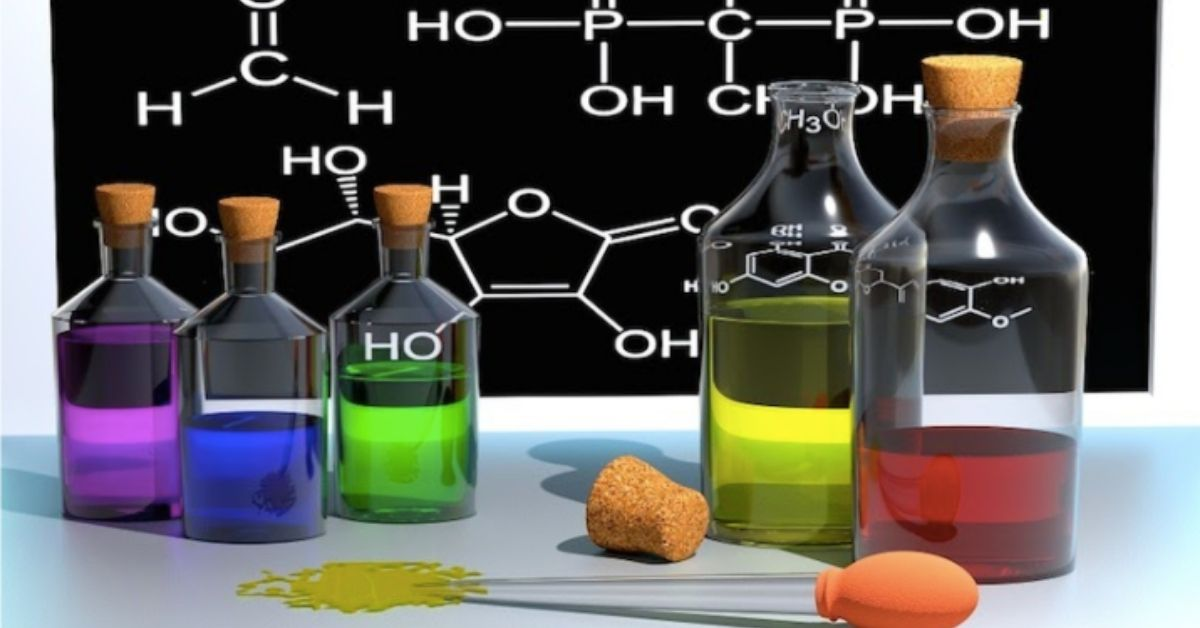# Becoming Much Smarter in ChemistrySteve Schecter
February 23, 2015

In my last post, I wrote about how students can use the Much Smarter method to overcome feelings of intimidation concerning science, and to begin mastering the game of biology. Today I’d like to extend the conversation to another domain of science: chemistry!

We generally understand chemistry to be the science concerning physical “matter,” the study of how things are made, how they combine, and how they come apart. When we think of chemistry, we often think of laboratories and of “hands-on” activities in which people mix stuff together and observe what happens!

Much of what students learn in high school chemistry class, however, is highly conceptual! You master chemistry by learning, and then using, models that describe how the physical world works. While these models have been validated by experimentation, you will not verify or visually observe most of them for yourself, and so, for you, these models will be conceptual.

Let me give you an example: students in most chemistry classes learn electron configuration, a model describing how those tiny particles are arranged in different kinds of atoms. Electron configuration explains an important way that elements differ from one another. Each element has a different number of electrons, and for each, the electrons are arranged differently.

You learn electron configuration as a conceptual model; you don’t look into an electron microscope and see the different arrangements of electrons, nor do you get to touch these electron arrangements.

The activities and skills that you need in order to master the game of chemistry are, for the most part, conceptual. Consider a few examples: using units of measure, converting one type of unit to another, using a quantity called “the mole,” balancing chemical equations, using chemical equations to predict the results of a chemical reaction. You master each of these activities by learning, and then using, a conceptual model.

For me, the best way to understand chemistry is to do chemistry. Going back to the example of electron configuration: when I merely thought about electron configuration, I found the concept abstract and difficult to comprehend. When I actively used the model of electron configuration to distinguish atoms of different elements, I “understood” the model.

In this respect, you can approach chemistry as you would math. Each of the moves we learn in the math game—adding fractions, multiplying exponents, solving an equation—requires us to learn and use a conceptual model. We get someone to show us the steps, we learn the steps ourselves, and then we practice the steps until we can perform the task effortlessly.

Approach chemistry the same way. For example, when it’s time to learn how to balance a chemical equation: 1) get someone (your chemistry teacher!) to show you the steps for accomplishing the task, 2) try the steps yourself, working slowly and deliberately until you can successfully perform the task once through, and then 3) practice the task until you can perform it effortlessly.

Take this same approach to all of the “moves” of the chemistry game. Learn each move one at a time, and then combine moves as you gain confidence and skill. This approach will help you both understand and perform the game of chemistry!